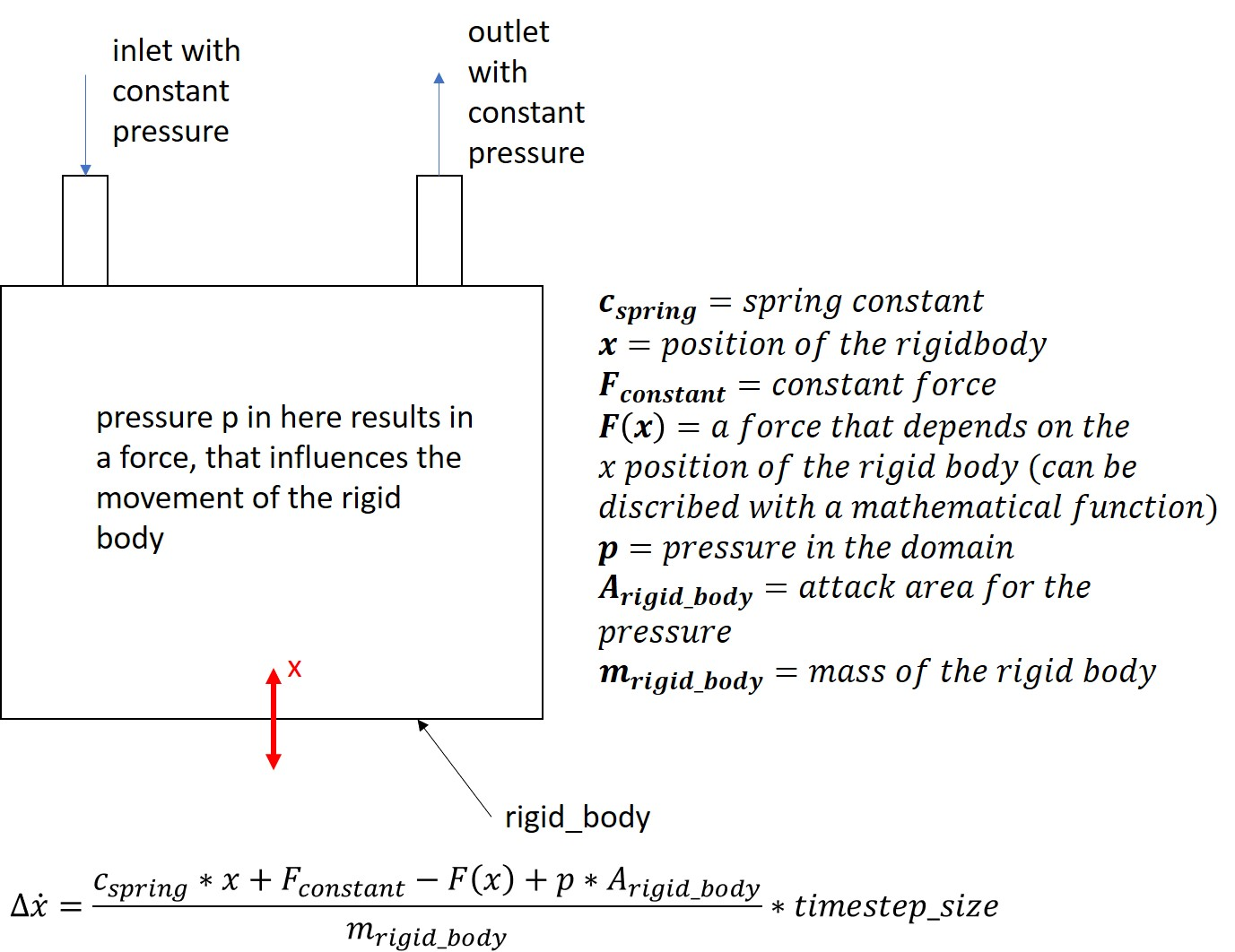# Defining Ansys Fluent UDF that defines the translation of a rigid body zoneHello guys,

I am faced to a problem where I have to simulate a rigid body movement with ANSYS 2020. The velocity of that rigid body is defined by various forces acting on the body. All these forces are shown in the picture. Inside the domain there is a pressure and together with the attack area of the rigid body the first force develops. What means that during the calculation for each timestep, Ansys has to include the "pressure force" acting on rigid body. Also there are other forces that are not directly seen in the model. For example there is a spring that depends on the position of the body. There is another force (F(x)) that depends on the position of the body. This dependency of force and position can be discribed by a mathematical function. If I sum up all these forces and divide them with the mass of that rigid body, we get the resulting acceleration for that rigid body. If we multiply that with the timestep size, we get a change in velocity for that particular timestep.

We also have to implement limits for the body`s movement. There is a upper and a lower limit, for each of them the velocity equals zero. The body movement starts if the balance of power is positive. As soon as the balance of power gets negative the body is decelerated and will move backwards or slower.

I think these physical connections can be somehow implemented by using the 6DOF solver, but we have to write a UDF for it, because of it's complexity. The big problem is, I haven't found any similar examples and/or good explanations that handel these kind of problems. And also I have no idea how the C programming language works.

Can you give me some hints or maybe an example how I can write this UDF file, so that I can go on with my work with that problem?

Thank you very much!